International
Tables for
Crystallography
Volume B
Reciprocal space
Edited by U. Shmueli

International Tables for Crystallography (2006). Vol. B, ch. 5.1, pp. 539-540   | 1 | 2 |

## Section 5.1.3.5. Pendellösung and extinction distances

A. Authiera*

aLaboratoire de Minéralogie-Cristallographie, Université P. et M. Curie, 4 Place Jussieu, F-75252 Paris CEDEX 05, France
Correspondence e-mail: authier@lmcp.jussieu.fr

#### 5.1.3.5. Pendellösung and extinction distances

| top | pdf |

This length plays a very important role in the dynamical theory of diffraction by both perfect and deformed crystals. For example, it is 15.3 µm for the 220 reflection of silicon, with Mo Kα radiation and a symmetric reflection.

In transmission geometry, it gives the period of the interference between the two excited wavefields which constitutes the Pendellösung effect first described by Ewald (1917)(see Section 5.1.6.3);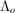in this case is called the Pendellösung distance, denoted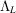hereafter. Its geometrical interpretation, in the zero-absorption case, is the inverse of the diameter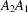of the dispersion surface in a direction defined by the cosines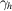and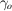with respect to the reflected and incident directions, respectively (Fig. 5.1.3.4). It reduces to the inverse of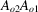(5.1.2.23)in the symmetric case.

In reflection geometry, it gives the absorption distance in the total-reflection domain and is called the extinction distance, denoted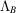(see Section 5.1.7.1). Its geometrical interpretation in the zero-absorption case is the inverse of the length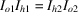, Fig. 5.1.3.5.

In a deformed crystal, if distortions are of the order of the width of the rocking curve over a distance, the crystal is considered to be slightly deformed, and ray theory (Penning & Polder, 1961; Kato, 1963, 1964a,b) can be used to describe the propagation of wavefields. If the distortions are larger, new wavefields may be generated by interbranch scattering (Authier & Balibar, 1970) and generalized dynamical diffraction theory such as that developed by Takagi (1962, 1969) should be used.

Using (5.1.3.8), expressions (5.1.3.5)and (5.1.3.6)can be rewritten in the very useful form: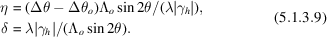The order of magnitude of the Darwin width 2δ ranges from a fraction of a second of an arc to ten or more seconds, and increases with increasing wavelength and increasing structure factor. For example, for the 220 reflection of silicon and Cu Kα radiation, it is 5.2 seconds.

### References

Authier, A. & Balibar, F. (1970). Création de nouveaux champs d'onde généralisés dus à la présence d'un objet diffractant. II. Cas d'un défaut isolé. Acta Cryst. A26, 647–654.Google Scholar
Ewald, P. P. (1917). Zur Begründung der Kristalloptik. III. Röntgenstrahlen. Ann. Phys. (Leipzig), 54, 519–597.Google Scholar
Kato, N. (1963). Pendellösung fringes in distorted crystals. I. Fermat's principle for Bloch waves. J. Phys. Soc. Jpn, 18, 1785–1791.Google Scholar
Kato, N. (1964a). Pendellösung fringes in distorted crystals. II. Application to two-beam cases. J. Phys. Soc. Jpn, 19, 67–77.Google Scholar
Kato, N. (1964b). Pendellösung fringes in distorted crystals. III. Application to homogeneously bent crystals. J. Phys. Soc. Jpn, 19, 971–985.Google Scholar
Penning, P. & Polder, D. (1961). Anomalous transmission of X-rays in elastically deformed crystals. Philips Res. Rep. 16, 419–440.Google Scholar
Takagi, S. (1962). Dynamical theory of diffraction applicable to crystals with any kind of small distortion. Acta Cryst. 15, 1311–1312.Google Scholar
Takagi, S. (1969). A dynamical theory of diffraction for a distorted crystal. J. Phys. Soc. Jpn, 26, 1239–1253.Google Scholar